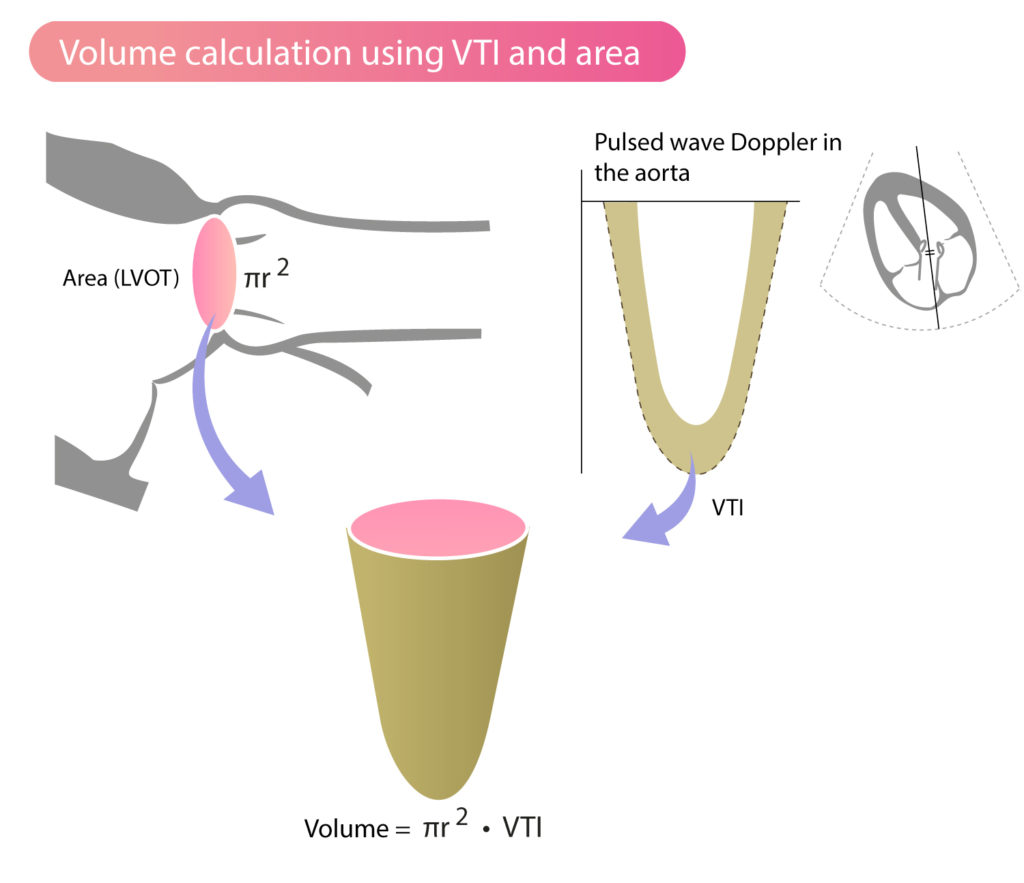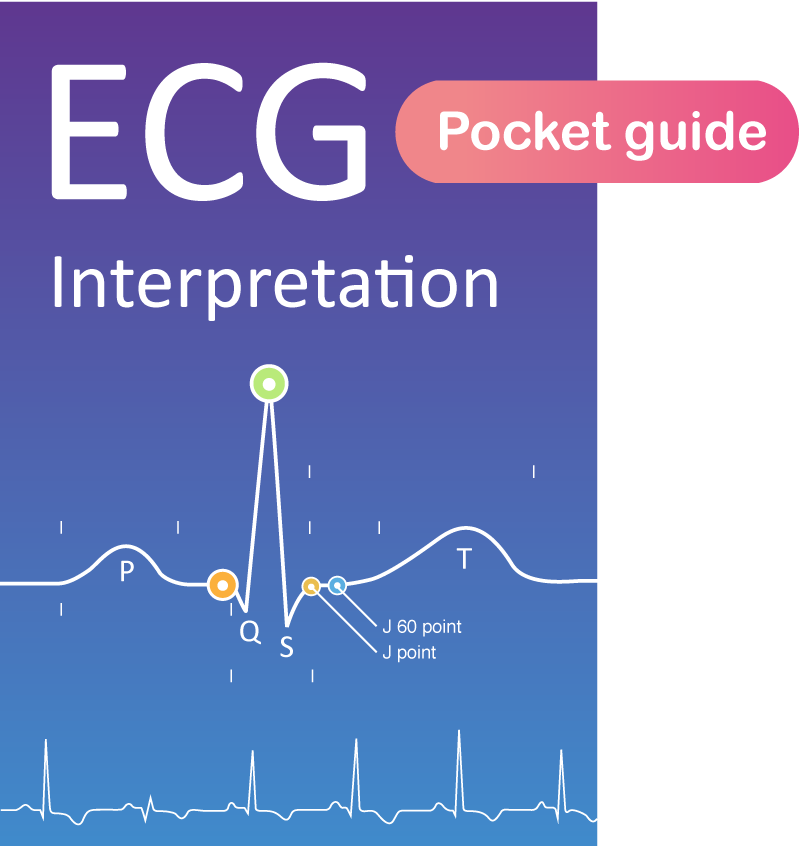Back to Book/course

## Clinical Echocardiography

0% Complete
0/0 Steps
Section 2, Chapter 5
In Progress

# Stroke Volume, VTI (Velocity Time Integral) & Cardiac Output

Chapter contents

Section Progress
0% Complete

## Principles of flows and volumes in the heart

If the flow in a cylinder is constant, then flow (Q) is the product of the cylinder area (a) and flow velocity (v):

Q = a • v

This principle can be used to estimate blood flow across the valves. As illustrated in Figure 1, the orifice of the aortic valve and the ascending aorta can be regarded as a cylinder, and the same assumption can be made for the other valves. The area is calculated by measuring the diameter of the valve (`area = π × radius2`, where `radius = diameter/2`), and velocity is measured by means of Doppler (Figure 1).

## Velocity Time Integral (VTI, stroke distance)

The formula `Q = a · v` states that flow (Q) is the product of area (a) of the cylinder and the velocity (v) of the fluid (i.e blood). The volume (V) that passes a specific segment is the product of the flow (Q) and time (t):

V = Q · t
V = volume; t = time (seconds).

However, this equation can only be used if the flow (Q) is constant, which is not the case in the heart. Blood flow is pulsatile during the cardiac cycle; flow is high during systole and ceases during diastole. Moreover, there are pronounced variations in flow during each phase, with rapidly accelerating flow in early systole, decelerating flow in late systole, and no flow during diastole. Doppler is capable of recording these flow variations with high precision.

To calculate flow across a valve, the Doppler line is placed in the valve orifice. The ultrasound machine displays the recorded flows as the spectral curve (Pulsed wave Doppler or Continuous wave Doppler). The area within the spectral curve is calculated automatically by the machine. This area is referred to as the VTI (Velocity Time Integral), and it measures how far blood travels during the time period. VTI is also called stroke distance. Figure 2 illustrates the recording of VTI using pulsed wave doppler in the aortic valve.

VTI (Velocity Time Integral) is the area within the spectral curve and indicates how far blood travels during the flow period. VTI can be used for various volume calculations, such as calculation of stroke volume.

## Stroke volume (SV), cardiac output (CO) and cardiac index (CI)

Stroke volume is the amount of blood ejected into the aorta during systole. Stroke volume is calculated by measuring the Doppler flow in the aortic valve. In the left ventricular outflow tract (LVOT), the following two measurements are performed:

• Diameter of the aortic annulus: This measurement is made in the parasternal long-axis view during systole, when the diameter is greatest (usually halfway through systole). Zoom in LVOT to improve the accuracy of the measurement.
• Flow velocity in LVOT: Velocity is measured in apical four-chamber view (4C) or five-chamber view (5C) using pulsed wave doppler with sample volume located in the valve orifice. The ultrasound machine calculates VTI (Velocity Time Integral) automatically.

This approach assumes that the valve orifice is approximately circular, such that the orifice area can be calculated using the diameter (`area = π × radius2`, where `radius = diameter/2`). The formula for stroke volume (SV) will then be:

SV = area • VTI
SV = stroke volume, VTI = velocity time integral.

Corresponding formula for measurements in the aortic valve:

SVaorta = areaLVOT • VTILVOT

The area is measured in cm2. VTI has the unit cm/contraction and stroke volume has the unit cm3/contraction (i.e ml/contraction). Figure 3 illustrates schematically how area and VTI are used to calculate stroke volume.Figure 3. Registration of VTI with pulsed wave doppler in the aortic opening.

## Cardiac output (CO)

Cardiac Output (L/min) is the product of stroke volume and heart rate:

CO = SV • HR
CO = cardiac output; SV = stroke volume; HR = heart rate.

## Cardiac Index (CI)

If cardiac output (CO) is divided by body surface area (BSA), then the Cardiac Index (L/min/m2) is obtained:

CI = CO / BSA
CI = cardiac index; CO = cardiac output; BSA = body surface area.

error: Contact us for permission to use contents. Permission will be granted for non-profit sites.

Join our newsletter and get our free ECG Pocket Guide!